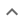•# Simplification or other simple results

We think you wrote:

###This solution deals with simplification or other simple results.

Solution found(y^4+6)*(3y-2)

## Step by Step Solution## Step  1  :

#### Equation at the end of step  1  :

  (((3 • (y5)) -  2y4) +  18y) -  12
Step  2  :

#### Equation at the end of step  2  :

  ((3y5 -  2y4) +  18y) -  12


## Step  3  :

#### Checking for a perfect cube :

3y5-2y4+18y-12  is not a perfect cube

#### Trying to factor by pulling out :

Factoring:  3y5-2y4+18y-12

Thoughtfully split the expression at hand into groups, each group having two terms :

Group 1:  18y-12
Group 2:  3y5-2y4

Pull out from each group separately :

Group 1:   (3y-2) • (6)
Group 2:   (3y-2) • (y4)
-------------------
Add up the two groups :
(3y-2)  •  (y4+6)
Which is the desired factorization

### Polynomial Roots Calculator :

Find roots (zeroes) of :       F(y) = y4+6
Polynomial Roots Calculator is a set of methods aimed at finding values of  y  for which   F(y)=0

Rational Roots Test is one of the above mentioned tools. It would only find Rational Roots that is numbers  y  which can be expressed as the quotient of two integers

The Rational Root Theorem states that if a polynomial zeroes for a rational number  P/Q   then  P  is a factor of the Trailing Constant and  Q  is a factor of the Leading Coefficient

In this case, the Leading Coefficient is  1  and the Trailing Constant is  6.

The factor(s) are:

of the Leading Coefficient :  1

of the Trailing Constant :  1 ,2 ,3 ,6

Let us test ....

P  Q  P/Q  F(P/Q)   Divisor
-1     1      -1.00      7.00
-2     1      -2.00      22.00
-3     1      -3.00      87.00
-6     1      -6.00      1302.00
1     1      1.00      7.00
2     1      2.00      22.00
3     1      3.00      87.00
6     1      6.00      1302.00

Polynomial Roots Calculator found no rational roots

## Final result :

  (y4 + 6) • (3y - 2)